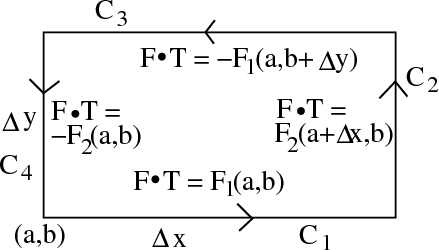# Math Insight

### Image: The vector field tangents used to derive a formula for the circulation per unit areaThe approximations for the vector field tangents along the rectangular curve $\dlc$ used in a step of the derivation of the circulation per unit area formula.